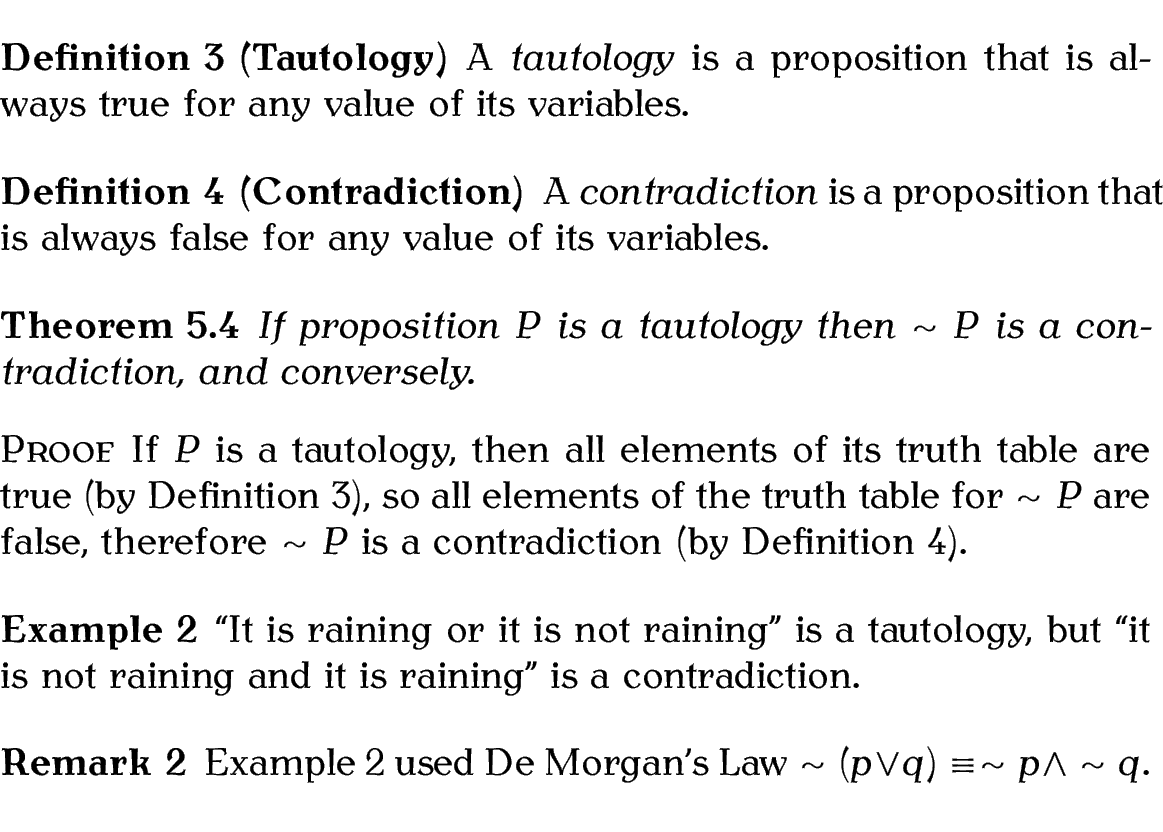## 4.7.2 The ntheorem Package

The ntheorem package provides nine predefined theorem styles, listed in Table 4.1. The default is plain. When you define a new theorem-like environment with \newtheorem, it is given the style currently in effect. You can change the current style with:

\theoremstyle{<style name>}

where <style name> is the name of the theorem style.

 plain Like the original LaTeX style break Header is followed by a line break change Like plain but header and number interchanged changebreak Combination of change and break margin Number is set in the margin marginbreak Like margin but header followed by a line break nonumberplain Like plain but without the number nonumberbreak Like break but without the number empty No number and no name. Only the optional argument is used in the header.

In addition to these styles, you can also use

\theoremheaderfont{<declarations>}

to set the header font to <declarations>, which should consist of font declaration commands such as \normalfont,

\theorembodyfont{<declarations>}

to set the body font to <declarations>, and

to set the appearance of the theorem number, where <style> may be one of: arabic, roman, Roman, alph, Alph, greek, Greek or fnsymbol. Remember that the above commands all need to be used before the new theorem-like environment is defined. For additional commands that affect the style of the theorems, see the ntheorem documentation .

Example:

% in the preamble:
\theoremstyle{marginbreak}
\theorembodyfont{\normalfont}
\newtheorem{note}{Note}[chapter]

% later in the document:
\begin{note}
This is a sample note. The number is in the margin.
\end{note}

Result (the vertical line in the image below indicates the boundary of the text area and won't appear in the PDF):If you use the standard package option to ntheorem, it will automatically define the following environments: Theorem, Lemma, Proposition, Corollary, Satz, Korollar, Definition, Example, Beispiel, Anmerkung, Bemerkung, Remark, Proof and Beweis.

Unlike amsthm's proof environment, ntheorem's Proof environment appends its optional argument in parentheses, if present, to the proof title. (Recall from earlier that amsthm's proof environment uses its optional argument as a replacement for the default proof title.)

Example:

% in the preamble:

\usepackage[standard]{ntheorem}

% later in the document:

\begin{Definition}[Tautology]\label{def:tautology}
A \emph{tautology} is a proposition that is always true for any value of its variables.
\end{Definition}

\begin{Definition}[Contradiction]\label{def:contradiction}
A \emph{contradiction} is a proposition that is always false for any value of its variables.
\end{Definition}

\begin{Theorem}
If proposition $P$ is a tautology then $\sim P$ is a contradiction, and conversely.
\begin{Proof}
If $P$ is a tautology, then all elements of its truth table are true (by Definition~\ref{def:tautology}), so all elements of the truth table for $\sim P$ are false, therefore $\sim P$ is a contradiction (by Definition~\ref{def:contradiction}).
\end{Proof}
\end{Theorem}

\begin{Example}\label{ex:rain}
It is raining or it is not raining'' is a tautology, but it is not raining and it is raining'' is a contradiction.
\end{Example}

\begin{Remark}
Example~\ref{ex:rain} used De Morgan's Law $\sim (p \vee q) \equiv \sim p \wedge \sim q$.
\end{Remark}

Result:This book is also available as A4 PDF or 12.8cm x 9.6cm PDF or paperback (ISBN 978-1-909440-02-9).

© 2013 Dickimaw Books. "Dickimaw", "Dickimaw Books" and the Dickimaw parrot logo are trademarks. The Dickimaw parrot was painted by Magdalene Pritchett.